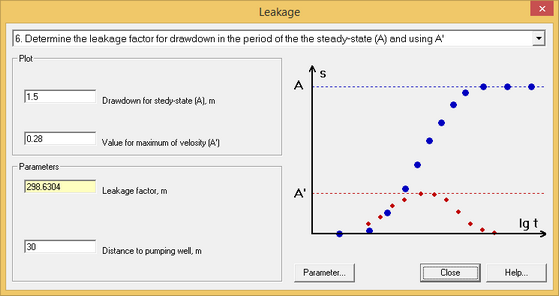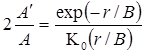﻿ Hydrogeologist workbench (ANSQUICK) > Estimation of parameters > Leakage factor

# Leakage factor

The dialog window "Leakage factor" is used for estimates of leaky factor and transmissivity in leaky aquifers (conceptual scheme: constant level in the adjacent aquifer).

The window is opened by "File > Hydrogeologist workbench > Estimation of parameters > Leakage factor".

This tool allows determination of factors/parameters that can be selected from the dropdown list. The dialog window contains only the parameters that are required for the item selected from the dropdown list.

1. Determination of leakage factor based on time of beginning of steady state regime ts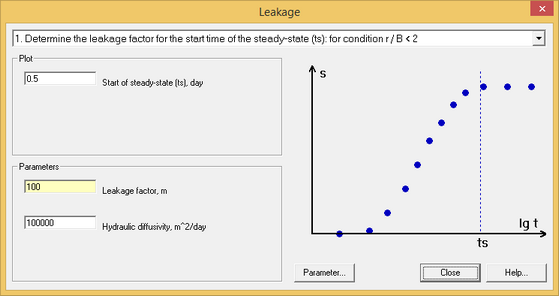The analytical solution exists only for the condition of r / B < 2.

For the above condition: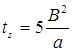and the calculated leakage factor is: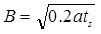2. Determines transmissivity based on the steady state drawdown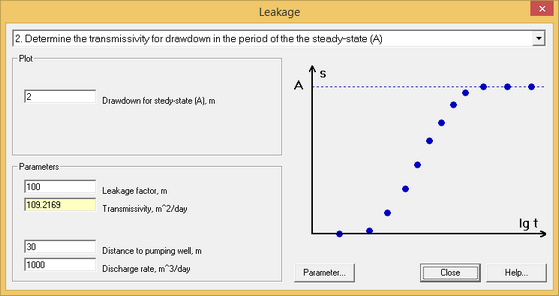Transmissivity is calculated using the maximum drawdown in observation well: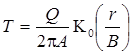Leakage factor is calculated as: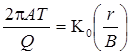3. Determines the leakage factor using time t', that corresponds to the maximum speed of drawdown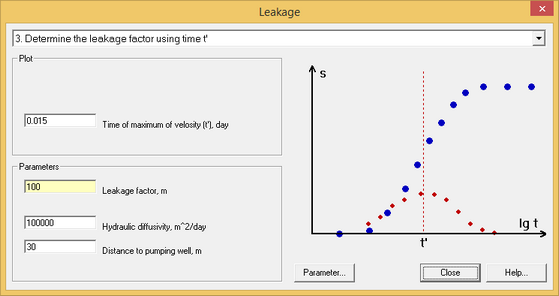This task is solved using a derivative analysis (red dots on the plot).

The time at which the maximum drawdown rate occurs during the pumping test is controlled by the leakage factor, hydraulic diffusivity and the distance between pumping and observation wells: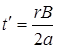From the above formula, the leakage factor is calculated as: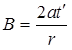The maximum speed of drawdown corresponds to the peak of the function, that is obtained by derivative analysis.

4. Determines transmissivity based on the maximum speed of drawdown.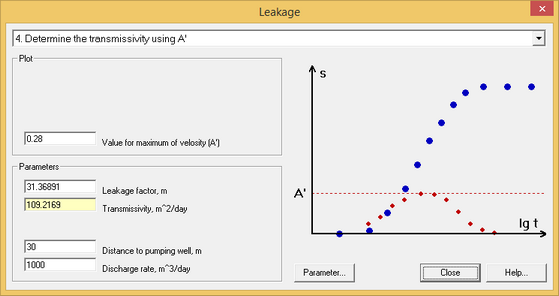This task is solved based on derivative analysis (see red dots on the plot).

Transmissivity is calculated by the following formula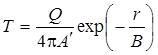When transmissivity is known, the leakage factor can be calculated as: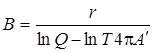5. Determines the leakage factor using the time ts of steady state and the time t' of maximum speed of drawdown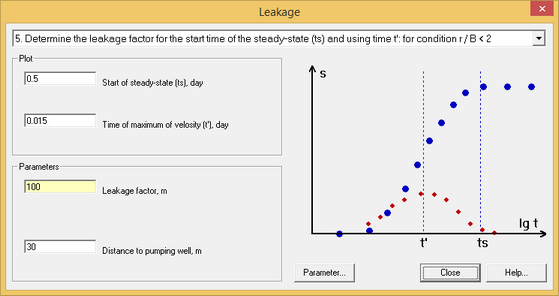This task is solved based on drawdown measurements (blue dots on the plot above) and results of the derivative analysis (red dots on the plot above). The solution assumes that: r / B < 2.

The leakage factor is calculated as: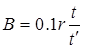6. Determines leakage factor using steady state drawdown and maximum speed of drawdown